Platform: All Platforms Versions: All versions

## Problem Description

How do I solve a PDE with space derivatives of order higher than two? For example, the equation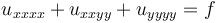for a function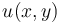.

## Solution

Introduce names for the second derivatives of u, say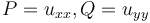.

Then the equation can be written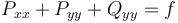.

You can now solve the following equivalent system of PDEs for the variables u, P, and Q with COMSOL Multiphysics: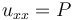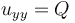This system can be entered as a General form PDE for the variables u, P, and Q, with the equations: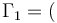`Px, Py + Qy`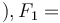`f`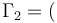`ux,0`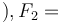`P`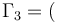`0,uy`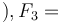`Q`

For boundary conditions, consider the following examples:
1. u, uxx, and uyy are given on the boundary. This can be implemented by using Dirichlet conditions for u, P, and Q.

1. u and its normal derivative du/dn are both given on the boundary. This implies that the derivative of u in the tangential direction can also be computed. Hence, expressions for ux and uy are known on the boundary. These boundary conditions can be implemented by using a Dirichlet condition for u, and Neumann conditions for P and Q: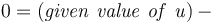`u`,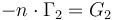,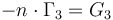,

where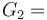-`nx`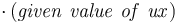and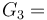-`ny`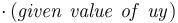.

The supplied example model solves this system of equations with f = 1 and the following boundary conditions:
Boundaries 1 and 2: u = 0, uxx = uyy = 0
Boundary 3: u = x, uxx = 0, uyy = -x
Boundary 4: u = sin(y), ux = sin(y), uy = cos(y)

Other equations of order higher than two can be treated similarly, by introducing names for certain derivatives of u. Use as high an order of Lagrange elements as you can afford, to make the higher order derivatives of u as smooth as possible.

## Related Files

 high_order_derivatives_53a.mph 281 KB